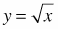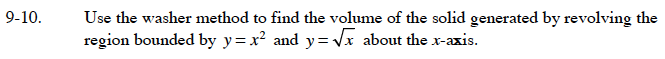### Home > CALC > Chapter 9 > Lesson 9.1.1 > Problem9-10

9-10.

Use the washer method to find the volume of the solid generated by revolving the region bounded by y = x2 andabout the x-axis. Homework Help ✎Start by determining the points of intersection. The x-values of the points of intersection will be the bounds of your integral (this is rotation about the x-axis, so the width of the washers is dx).

The general formula for the washer method is:

Which function is the outer radius R and which function is the inner radius r?